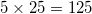# ISEE Lower Level Math : Rectangles

## Example Questions

← Previous 1 3 4 5 6 7 8 9 10 11 12

### Example Question #11 : Plane Geometry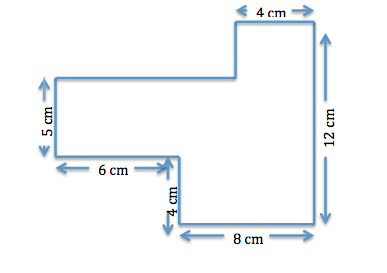What is the total surface area of the enclosed region?

114cm2

168cm2

212cm2

112cm2

126cm2

114cm2

Explanation: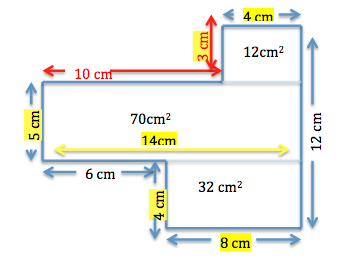First, we must find the missing lengths. Because we know that the length going horizontally across on the bottom is 6 cm and 8 cm, that must mean that the length going across at the top must also equal this sum.

So, the length of the top must equal

6 + 8 =

14.

We are given one value of the length on the top, 4. To find the missing horizontal length on the top, we must subtract 4 from 14.

14 – 4 = 10.

In order to find the other missing length, we must observe that the greatest vertical length of this figure is 12 cm. Because we are given 4 cm and 5 cm, we must subtract 4 and 5 from 12 to find the other missing length.

12 – 4 – 5 =

3.

Now, let's divide this enclosed region in three separate rectangles.

The rectangle at the top has a length of 4 cm and width of 3 cm.

The middle rectangle has a length of

10 + 4 =

14 cm

and a width of 5 cm.

The bottom rectangle has a length of 8 cm and width of 4 cm.

If the formula for the area of a rectangle is length x width, we must now calculate the individual areas of each rectangle and add them up.

Area of top rectangle

4 x 3 = 12cm2

Area of middle rectangle

14 x 5 = 70cm2

Area of bottom rectangle

8 x 4 = 32cm2

12cm2 + 32cm2 + 70cm=

114cm2

### Example Question #1 : How To Find The Area Of A Rectangle

Mr. Barker is building a rectangular fence. His yard has an area of 24 feet, and the one side of the fence he's already built is 6 feet long.

What is the length of the other side (the width) of the fence?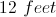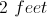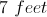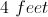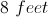Explanation:

The answer is 4 feet, because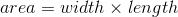and a rectangle must have 4 sides, with 2 sides of one length and 2 sides of another.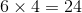feet

making the other side 4 feet,

### Example Question #12 : Plane Geometry

If Bailey is making a quilt for a bed that measuresfeet wide andfeet long, and she wants there to be an extra foot of quilt to hang over each side of the bed, how much material should she buy.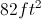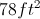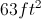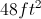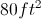Explanation:

In order to determine how much material Bailey needs, we must first find the area of space she needs to cover. Since Bailey would like the quilt to be an extra foot on each side, we must addfeet to both the length and width.

Width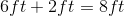Length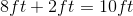Now we apply the formula for the area of a rectangle, which is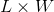.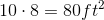### Example Question #1 : How To Find The Area Of A Rectangle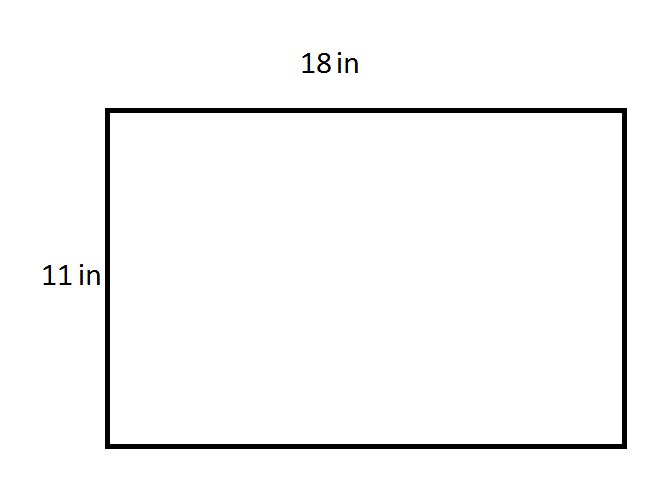Give the area of the rectangle in the above diagram.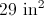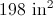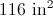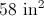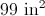Explanation:

Multiply the length by the width to get the area of the rectangle: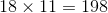The area of the rectangle is 198 square inches.

### Example Question #5 : How To Find The Area Of A Rectangle

Which could be the dimensions of a rectangle with the area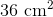?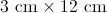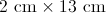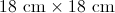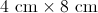Explanation:

To find the area, simply multiply the sides of the rectangle. The only sides which add up toare: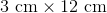### Example Question #2 : How To Find The Area Of A Rectangle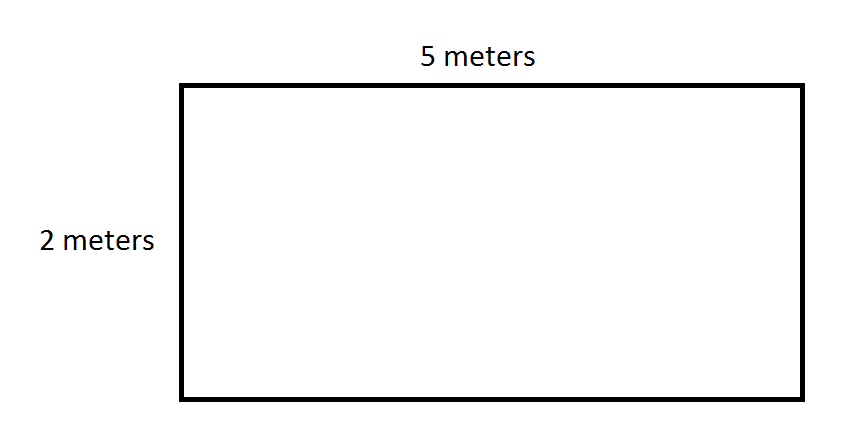Give the area of the above rectangle in square centimeters.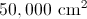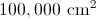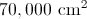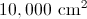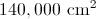Explanation:

Since 1 meter = 100 centimeters, multiply each dimension by 100 to convert meters to centimeters. This makes the dimensions 200 centimeters by 500 centimeters.

Use the area formula, substituting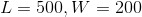: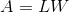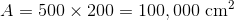### Example Question #2 : How To Find The Area Of A Rectangle

Mr. Smith is planting a rectangular garden with a length of 5 ft and a width of 7 ft. What is the area of the garden?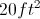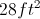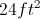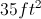Explanation:

The area of a rectangle can be calculated by multiplying the length by the width.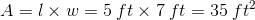### Example Question #8 : How To Find The Area Of A Rectangle

Sally's room measures 100 square feet. Which type of measurement is this?

Perimeter

Length

Area

Volume

Width

Area

Explanation:

Area is measured in units squared, and volume is measured in units cubed. Width, length, and perimeter are all measured in regular units.

Therefore, 100 square feet is the area of the room.

### Example Question #2 : How To Find The Area Of A Rectangle

Robert needs to determine the area of his rectangular wall in order to buy the proper amount of paint at the store. When he measures his wall he determines that it is 12 feet high and 20 feet wide. If one can of paint covers 40 square feet of wall, how many cans of paint does Robert need?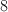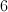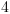Explanation:

Find the area of the wall -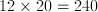Divide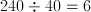6 cans of paint.

### Example Question #2 : How To Find The Area Of A Rectangle

What is the area of a rectangle with a length offeet and a width offeet?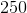square feetsquare feetsquare feetsquare feetsquare feetsquare feet